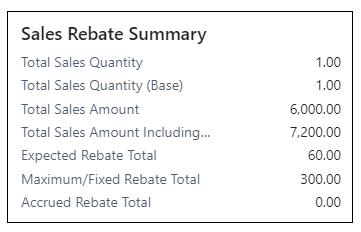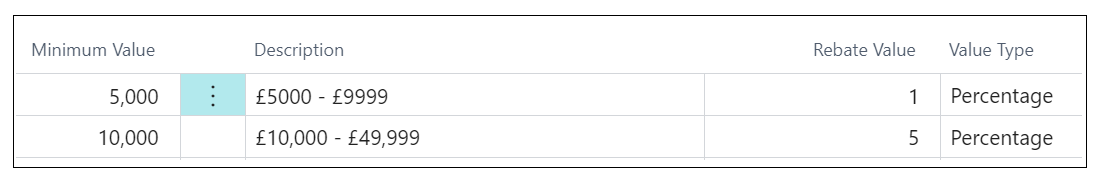On the Rebate Card, you will see a Sales/Purchase Rebate Summary FactBox such as the below image which gives you a quick overview regarding the current rebate status for the Customer or Vendor. This records Total Quantity (where the rebate is being calculated by Quantity invoiced), Total Quantity Base (where the rebate is being calculated by Quantity Base (UoM)) and Total Amount to calculate rebates using 'Percentage'. Although the Total Amount including VAT is mentioned, it is not used to calculate any rebates, it is there for information only.Also available here is the Expected Rebate Total. This is a sum of all of the "Rebate Entries" based on what rebate value has actually been achieved so far, so for example if using "Scheme Steps", even where I had a tier for a 5% Rebate value, the Rebate Summary Total Amount is only for '£6000' which only incurs a 1% rebate. Thus the Expected Rebate Total would display the total rebate value based on 1% because this is what that Customer has achieved within this rebate so far.Beneath this is the Maximum/Fixed Rebate Total. This will detail the value for this Rebate Card based on what the maximum rebate value will be for the accruals, using the value you have stored in the Maximum/Fixed Rebate field. So for example in the Sales Rebate Summary image above, the Maximum/Fixed Rebate Total is using my Maximum/Fixed Rebate value of '5' and using this as a percent (as in this example, the Value Type is 'Percentage') of the Total Sales Amount to show the highest amount that will be used for the accrual. As my Total Sales Amount is '£6000', 5% of this is '£300'. This is here just as a quick view to see how much will be accrued for.

Finally the Accrued Rebate Total is the physical amount that has been accrued so far for this Rebate Card. This is calculated as soon as the Accrual process has been ran and will add Accrual Amounts to the "Rebate Entries", using these values per entry as a sum to calculate this field. More information on the accrual amounts can be found here. For any accruals that are re-opened, the value re-opened will be deducted from the Accrual Amount value as this shows what has been Accrued only.